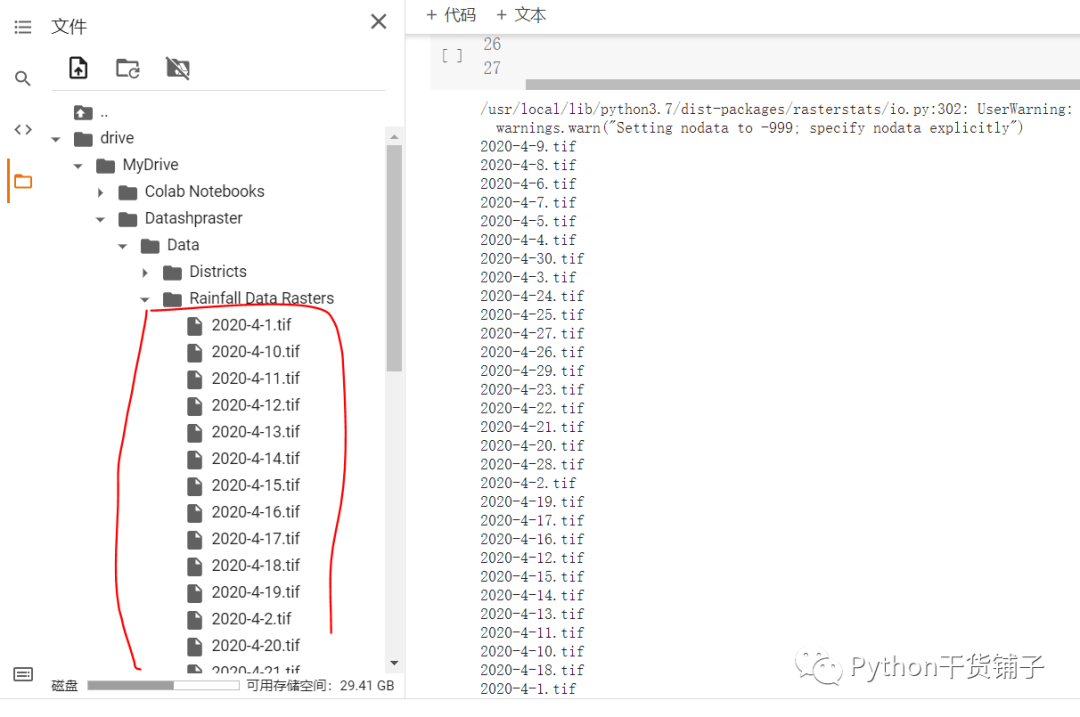2021
07-21

# 用Python进行栅格数据的分区统计和批量提取```import geopandas as gpd
import pandas as pd
import numpy as np
import matplotlib.pyplot as plt
import rasterio
import rasterstats
from rasterio.plot import show
# show()方法用来展示栅格图形
from rasterio.plot import show_hist
# 用来展示直方图
import cartopy.crs as ccrs
import cartopy.feature as cfeature
from cartopy.mpl.ticker import LongitudeFormatter, LatitudeFormatter```

```# 使用geopandas读取矢量数据

# 使用rasterio读取栅格数据，栅格数据和矢量数据的坐标投影需要一致
raster = rasterio.open('/content/drive/MyDrive/Datashpraster/Data/Rainfall Data Rasters/2020-4-1.tif')```
```# 把矢量数据和栅格数据绘制到一个axis上，这个axis不是坐标轴，而是图形
plt.rcParams['font.family'] = 'Times New Roman'
plt.rcParams['font.size'] = 20

fig, (ax1,ax2) = plt.subplots(1,2,figsize=(15,6))

show(raster, ax=ax1,title='Rainfall')
# 读取进来的矢量数据可以直接调用gpd的plot()方法绘制
districts.plot(ax=ax1, facecolor='None', edgecolor='red')
show_hist(raster,ax=ax2,title='hist')

plt.show()``````# 提取雨量栅格值到numpy数组
# 遵循GDAL规则从第一波段读取
rainfall_data``````# 设置坐标变换信息
affine = raster.transform

# 准备开始进行空间分区计算
# 第一个参数是矢量分区，第二个是栅格，第三个是坐标变换信息，第四个是统计均值
avg_rallrain = rasterstats.zonal_stats(districts,rainfall_data,affine=affine,stats=['mean'],geojson_out=True)
# avg_rallrain

# 除了统计平均值之外，还有最大最小值那些```，就是这些tif数据loop这些栅格数据集：```# 将Date列转为时间型
data['Date'] = pd.to_datetime(data['Date'], infer_datetime_format=True)

# print(data)

data['Date'] = data['Date'].dt.date
print(data)``````# 准备绘制图形
fig,(ax1,ax2)= plt.subplots(2,1,figsize=(18,6))
plt.rcParams['font.size'] = 15

data.plot(x='Date', y='Average_RF_Porto', ax=ax1, kind='bar', title='Avg_Rail_Porto')
data.plot(x='Date', y='Average_RF_Faro', ax=ax2, kind='bar', title='Avg_Rail_Faro',color='red')

#自动调整图形的分布
plt.tight_layout()
plt.show()```